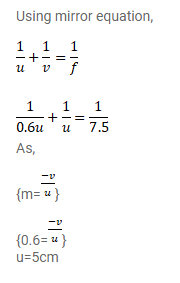# A 1cm object is placed perpendicular to the principalQuestion:

A $1 \mathrm{~cm}$ object is placed perpendicular to the principal axis of a convex mirror of focal length $7.5 \mathrm{~cm}$. Find its distance from the mirror if the image formed is $0.6 \mathrm{~cm}$ in size.

Solution: